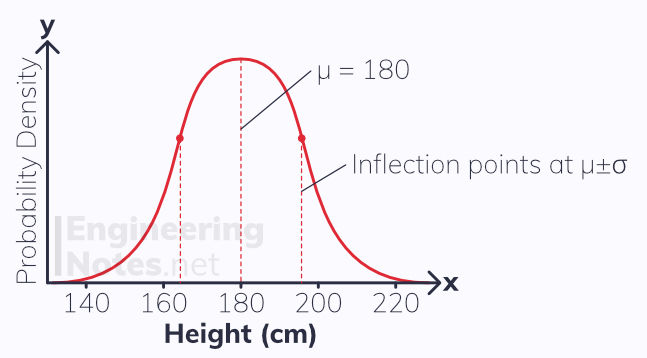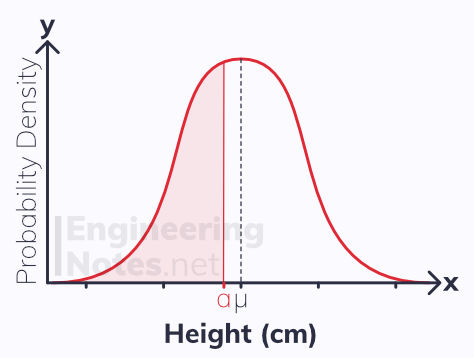top of page

#### Notes by Category University Engineering

Rate these notesNot a fanNot so goodGoodVery goodBrillRate these notes

# Statistical Distributions

A random variable is a variable whose value is dependent on the outcome of a random event. This means the value is not known until the experiment is carried out, however the probabilities of all the outcomes can be modelled with a statistical distribution.

There are multiple ways of writing out a distribution:The examples above are all the distribution of a fair, six-sided die. The probability of each outcome is the same, so it is called a discrete uniform distribution.

The sum of all probabilities in a distribution must equal 1

## The Binomial Distribution

If you repeat an experiment multiples times (each time is known as a 'trial'), you can model the number of successful trials with the random variable, X.

A binomial distribution is used when:

• There are a fixed number of trials, n

• There are only two possible outcomes (success or fail)

• The probability, p, of success is constant

• All trials are independent

If the random variable X is distributed binomially with n number of trials and fixed probability of success p, it is noted as:

X∼B(n, p)

Generally, you use a calculator to calculate binomial problems, but you can also use the probability mass function:There are two forms of the binomial distribution: exact and cumulative:

### Exact Binomial Problems

This is when a question asks you to find the probability of there being a specific number of successes out of the number of trials, n.

For example, to find the probability of there being exactly 4 successes for the random variable X∼B(12, 0.4) use an exact binomial distribution.

To find this on a CASIO ClassWix fx-991EX:

2. Click 7: Distribution

3. Click 4: Binomial PD

4. Click 2: Variable

5. Input your values - in this example, x=4, N=12, p=0.4

6. Click =

You should find the answer for this example is 0.213. This means there is a 21.3% chance of getting exactly four successful trials, out of a total 12 trials.

Using the table function allows you to see the probabilities for multiple values of x.

### Cumulative Binomial Problems

Generally, this is used more, and is used to find the sum of all the probabilities up to and including a certain value of x: P(X ≤ x)

For example, if you want to find the probability of there being up to and including 4 successes (so there could be 0, 1, 2, 3 or 4 successes) for the random variable X∼B(12, 0.4), use the cumulative binomial distribution. This can be done in two ways:

### Tables

There are tables with values for this, typically found at the back of formula books. These will have the most commons values for n, and some standard probabilities.

### Calculators

To find this on a CASIO ClassWix fx-991EX:

2. Click 7: Distribution

3. Click DOWN

4. Click 1: Binomial CD

5. Click 2: Variable

6. Input your values - in this example, x=4, N=12, p=0.4

7. Click =

Tables and calculators only ever give the probability for 'up to and including x', P(X ≤ x)

Therefore, if you want other forms, such as P(X > a) you need to use the following functions:

• For P(X > a), use 1 - P(X ≤ a)

• For P(X < a), use P(X ≤ (a-1))

• For P(X ≥ a), use 1 - P(X ≤ (a-1))

• For P(X ≤ a), use P(X ≤ a)

These rules work because the sum of all the probabilities equals 1.

## The Normal Distribution

The normal distribution is used to model continuous random variables. These are variables that can take absolutely any value.

The probability that the continuous random variable takes a particular specific value is always zero, but we can calculate the probability that it takes a value within a certain range. This is because continuous random variables have a continuous probability distribution:

• It is modelled as a curved graph

• The probability is the area under the curve

• The area under the curve can only be defined for ranges, as the area of an infinitely narrow line is zeroBecause the area under the graph is the probability, and the sum of all probabilities is 1, the area under the whole graph = 1

You can think of a continuous probability distribution as a histogram with an infinite number of infinitely narrow categories:### The Normal Distribution

A normal distribution is a continuous probability distribution that is bell-shaped and symmetrical about the mean.

• μ is the population mean, and is in the middle of the distribution

• σ is the standard deviation of the population

• σ² is the population variance

• The graph is symmetrical about the mean

• The graph has a total area of 1

• There are points on inflection at μ + σ and μ - σ

If the continuous random variable X is distributed normally with population mean μ and standard deviation σ, it is noted as:

X∼N(μ, σ²)All things in nature tend to be modelled with a normal distribution (hence the name), especially heights and lengths of members of a population.

It is good to know how the data is spread across the graph:

• Around 68% of all data is within one standard deviation of the mean (between the two points of inflection)

• Around 95% of all data is within two standard deviations of the mean

• Around 99.7% of all data is within three standard deviations of the mean

### Example

In the example above, the median is 180 cm, and the standard deviation is 16. Therefore, continuous random variable, X, is modelled: X∼N(180, 16²). Find P(170 < X < 190)

To find this on a CASIO ClassWix fx-991EX:

2. Click 7: Distribution

3. Click 2: Normal CD

4. Input your values - in this example, lower=170, upper = 190, σ=16, μ=180

5. Click =

You should find the answer for this example is 0.468. This means there is a 46.8% chance of someone's height being between 170 cm and 190 cm.

If only one boundary is specified, e.g. P(X < 190) or P(X > 170), make the other boundary a ridiculously big negative or positive number.
• For P(X < 190), the upper boundary is 190, and make the lower one -9999999999999999 or something similar

• For P(X > 170), the lower boundary is 170 and make the upper one 9999999999999999 or something similar

### Inverse Normal

You can also use the normal distribution backwards, to find limits from probabilities. This is done using the inverse normal distribution function.

Continuing with the example above, where X∼N(180, 16²): find the value of a for which P(X < a) = 0.35.

It is sometimes useful to represent this visually:To find this on a CASIO ClassWix fx-991EX:

2. Click 7: Distribution

3. Click 3: Inverse Normal

4. Input your values - in this example, area=0.35 σ=16, μ=180

5. Click =

You should find the answer for this example is 174. This means there is a 35% chance of someone's height being between less than 174 cm

Calculators only ever calculate the area to the left
• This means that if you want to find the value of a for which P(X > a) = 0.35, you need to input 0.65 (1-0.35) into your calculator.

### Standard Normal

Normally distributed variables can be standardised using coding:

The standard normal distribution has mean 0 and standard deviation 1

If X∼N(μ, σ²), it can be coded into Z∼N(0, 1²) using the equation Z = (X-μ) / σ• Sometimes, the probability P(Z < a) is written as Φ(a)

You use your calculator normally, just enter μ = 0, σ = 1.

### Finding μ and σ

Often, you will not know either the mean or the standard deviation of a normal distribution and will have to find it. You will, however, be given a probability, so you can code it into the standard normal distribution and solve.

For example, the random variable X ∼ N(μ, 3²). Given that P(X< 10) = 0.3, find the mean.

• Use the inverse normal to find the value for Z when p = 0.3: